Popularity
9.0
Stable
Activity
0.0
Stable
82
10
10

# unm-hip alternatives and similar packages

Based on the "Image Processing" category.
Alternatively, view unm-hip alternatives based on common mentions on social networks and blogs.

• ### picedit

simple set of functions and cli for image manipulation
• ### latex-svg-hakyll

Use actual LaTeX to render formulae inside Hakyll pages
• ### Collect and Analyze Billions of Data Points in Real Time

Manage all types of time series data in a single, purpose-built database. Run at any scale in any environment in the cloud, on-premises, or at the edge.
• ### latex-svg-image

A library for rendering LaTeX formulae as SVG using an actual LaTeX
• ### latex-svg-pandoc

Render LaTeX formulae in pandoc documents to images with an actual LaTeX

Do you think we are missing an alternative of unm-hip or a related project?

## University of New Mexico's Haskell Image Processing Library

To get started, import `Data.Image` or `Data.Image.Boxed`. To use unm-hip interactively in ghci, import `Data.Image.Interactive`. This provides three useful functions: `display`, `setDisplayProgram`, and `plotHistograms`.

``````setDisplayProgram :: String -> Bool -> IO ()
``````

Sets the program to use when making a call to `display` and specifies if the program can accept an image via stdin. If it cannot, then a temporary file will be created and passed as an argument instead. By default, ImageMagick (`display`) is the default program to use and it is read using stdin.

``````*Main> setDisplayProgram "gimp" False
*Main> setDisplayProgram "xv" False
*Main> setDisplayProgram "display" True

display :: DisplayFormat df => df -> IO (Handle, Handle, Handle, ProcessHandle)
``````

Makes a call to the current display program to be displayed. If the program cannot read from standard in, a file named .tmp-img is created and used as an argument to the program.

``````makeImage :: Image i => Int -> Int -> PixelOp (Pixel i) -> i
``````

Given an `Int` m, `Int` n, and a `PixelOp` f, `makeImage` returns an Image with dimensions m x n and the `Pixel` value at each (i, j) is `f i j`.

``````*Main> let grad = makeImage 128 128 (\ r c -> fromIntegral (r + c)) :: GrayImage
< Image 128x128 >
``````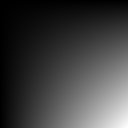``````pii :: Complex Double
pii = 0 :+ pi

harmonicSignal :: Double -> Double -> Int -> Int -> Complex Double
harmonicSignal u v m n = exp ((2*pii) * ((u*(fromIntegral m) + v*(fromIntegral n)) :+ 0))

*Main> let signal = makeImage 128 128 (harmonicSignal (3 / 128) (2 / 128)) :: ComplexImage
*Main> signal
*Main> signal
< Image 128x128 >
*Main> display signal
``````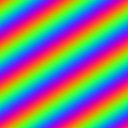``````readImage :: FilePath -> IO GrayImage
``````

Given the file path to a file containing an image stored in ASCII .pgm format, `readImage` reads the file and returns the `Image`. For example,

``````*Main> frog &lt;- readImage "images/frog.pgm"
*Main> display frog
``````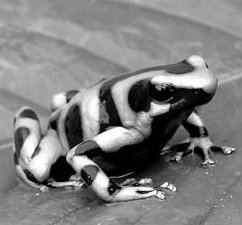``````writeImage :: DisplayFormat df => FilePath -> df -> IO ()
``````

Given a filename and an Image, `writeImage` creates a file representing the image in ASCII .pgm format for `GrayImage`s and .ppm for `ColorImage` and `ComplexImage`. Note: Images saved this way are normalized to integers in the range 0 to 255; this may result in loss of detail.

``````*Main> writeImage "frog.pgm" frog
``````

creates a file which looks like this:

``````P2
242 225
255
151   151   151   151   151   150   150   149   148   147   146   145   145   142   142
143   145   148   152   156   158   159   159   159   159   157   155   152   150   153
152   151   149   149   149   149   150   149   149   149   149   149   149   149   149
149   146   144   141   138   136   133   132   136   136   136   136   136   136   136
136   139   138   138   138   137   136   136   136   135   135   136   136   137   137
138   138   138   137   138   137   138   137   138   137   135   134   134   134   138
141   147   150   149   147   143   138   134   132   131   130   129   129   130   132
134   136   137   137   137   137   138   139   142   145   147   149   145   146   150
153   156   159   161   163   156   158   161   163   167   170   174   175   181   183
.
.
.
``````
``````ref :: Image i => i -> Int -> Int -> Pixel i
``````

Given an image, a positive `Int` i, and a positive `Int` j, `ref` returns the `Pixel` value at location (i, j).

``````*Main> ref frog 100 100
56.0
``````
``````ref' :: ref' :: GrayImage -> Double -> Double -> Double
``````

Given a `GrayImage`, a positive `Double` i, and a positive `Double` j, `ref'` returns the bilinear interpolated `Pixel` value at location (i, j).

``````*Main> ref' frog 100 100
56.0
``````
``````rows :: Image i => i -> Int
``````

Given an `Image`, `rows` returns the number of rows of in the `Image`.

For example,

``````*Main> rows frog
225
``````
``````cols :: Image i => i -> Int
``````

Given an `Image`, `cols` returns the number of columns of in the `Image`.

For example,

``````*Main> cols frog
242
``````
``````transpose :: Image img => img -> img
``````

Given an `Image` img, `transpose` returns an `Image` created by interchanging the rows and columns of the `Image`, i.e., the value at location (i, j) of the result `Image` is the value of the img at location (j, i).

For example,

``````*Main> transpose frog
< Image 242x225 >
*Main> display . transpose \$ frog
``````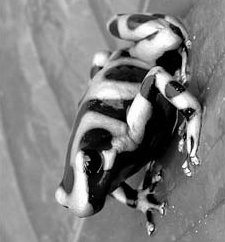``````convolveRows :: (Num (Pixel img), Image img) => [Pixel img] -> img -> img
``````

Given a list consisting solely of `Pixel` values representing a 1D convolution kernel and an `Image`, `convolveRows` returns the 1D discrete periodic convolution of the rows of the `Image` with the kernel.

For example,

``````*Main> convolveRows [1, -1] frog
< Image 225x242 >
*Main> display . convolveRows [1, -1] \$ frog
``````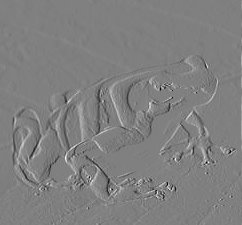``````convolveCols :: (Num (Pixel img), Image img) => [Pixel img] -> img -> img
``````

Given a list consisting solely of `Pixel` values representing a 1D convolution kernel and an `Image`, `convolveCols` returns the 1D discrete periodic convolution of the columns of the `Image` with the kernel.

For example,

``````*Main> convolveCols [1, -1] frog
< Image 225x242 >
*Main> display . convolveCols [1, -1] \$ frog
``````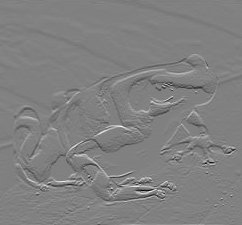``````*Main> let dx = convolveRows [1, -1] frog
*Main> let dy = convolveCols [1, -1] frog
*Main> let grad = imageMap sqrt ((dx * dx) + (dy * dy)) :: GrayImage
< Image 225x242 >
``````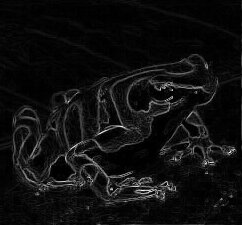``````convolve :: (Num (Pixel img), Image img) => \[\[Pixel img\]\] -> img -> img
``````

Given a 2D list consisting solely of `Pixel`s representing a 2D convolution kernel and an `Image`, `convolve` returns the 2D discrete periodic convolution of the `Image` with the kernel.

For example,

``````*Main> convolve [[1, 1, 1], [1, -8, 1], [1, 1, 1]] frog
< Image 225x242 >
*Main> display . convolve [[1, 1, 1], [1, -8, 1], [1, 1, 1]] \$ frog
``````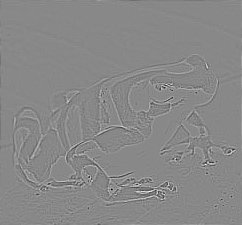``````downsampleCols :: Image img => img -> img
``````

Given img, `downsampleCols` returns the image created by discarding the odd numbered rows, i.e., the value at location (i, j) of the result image is the value of img at location (2i, j).

For example,

``````*Main> downsampleCols frog
< Image 112x242 >
*Main> display . downsampleCols \$ frog
``````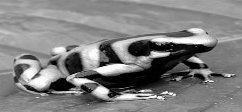``````downsampleRows :: Image img => img -> img
``````

Given img, `downsampleRows` returns the image created by discarding the odd numbered columns, i.e., the value at location (i, j) is the value of img at location (i, 2j).

For example,

``````*Main> downsampleRows frog
< Image 225x121 >
*Main> display . downsampleRows \$ frog
``````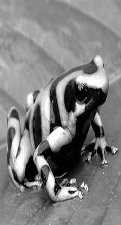``````downsample :: Image img => img -> img

*Main> let tinyFrog = downsample frog
*Main> tinyFrog
< Image 112x121 >
*Main> display tinyFrog
``````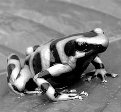``````upsampleCols :: (Monoid (Pixel img), Image img) => img -> img
``````

Given img, `upsampleCols` returns an `Image` with twice the number of rows where the value at location (i, j) of the result `Image` is the value of img at location (i/2, j) if i is even and `mempty` otherwise.

For example,

``````*Main> upsampleCols tinyFrog
< Image 224x121 >
*Main> display . upsampleCols \$ tinyFrog
````````````upsampleRows :: (Monoid (Pixel img), Image img) => img -> img
``````

Given img, `upsampleRows` returns an `Image` with twice the number of columns where the value at location (i, j) of the result `Image` is the value of img at location (i, j/2) if j is even and `mempty` otherwise.

For example,

``````*Main> upsampleRows tinyFrog
< Image 112x242 >
*Main> display . upsampleRows \$ tinyFrog
``````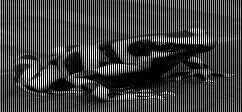``````upsample :: (Monoid (Pixel img), Image img) => img -> img
``````

Given img, `upsample` returns an`Image` with twice the number ofrows and columns where the value at location (i, j) of the resulting image is the value of img at location (i/2, j/2) if i and j are are even and `mempty` otherwise.

For example,

``````*Main> upsample tinyFrog
< Image 224x242 >
*Main> display . upsample \$ tinyFrog
``````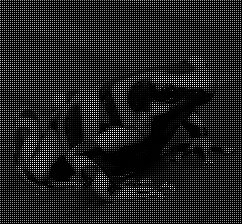``````pad :: (Monoid (Pixel img), Image img) => Int -> Int -> img -> img
``````

Given m, n, and img, `pad` returns an `Image` with m rows and n columns where the value at location (i, j) of the result `Image` is the value of img at location (i, j) if i is less than m and j is less than n. and `mempty` otherwise.

For example,

``````*Main> pad 200 200 tinyFrog
< Image 200x200 >
*Main> display . pad 200 200 \$ tinyFrog
``````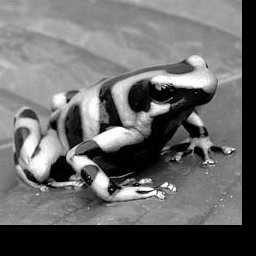``````crop :: Image img => Int -> Int -> Int -> Int -> img -> img
``````

Given a i0, j0, m, n, and img, `crop` returns an `Image` with m rows and n columns where the value at location (i, j) of the result `Image` is the value of img at location (i0 + i, j0 + j).

For example,

``````*Main> let frogPart = crop 64 64 128 128 frog
*Main> frogPart
< Image 128x128 >
*Main> display frogPart
``````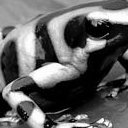``````leftToRight :: Image img => img -> img -> img<
``````

Given two `Images` with the same number of rows X and Y, `leftToRight` returns an `Image` that is the concatenation of the two `Images` from left to right. There is a convenience function, `leftToRight'` that takes a pair, triple, or list of `Images` and displays them left to right.

For example,

``````*Main> leftToRight tinyFrog tinyFrog
< Image 112x242 >
*Main> display . leftToRight tinyFrog \$ tinyFrog
``````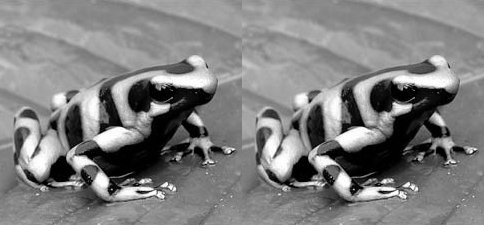``````topToBottom :: Image img => img -> img -> img
``````

Given two `Images` with the same number of columns X and Y, `topToBottom` returns an image that is the concatenation of the two images from top to bottom. There is a convenience function, topToBottom' that takes a pair, triple, or list of `Images` and displays them top to bottom.

For example,

``````*Main> topToBottom tinyFrog tinyFrog
< Image 224x121 >
*Main> display . topToBottom tinyFrog \$ tinyFrog
``````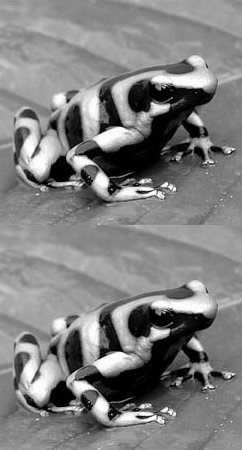``````makeFilter :: Image img => Int -> Int -> PixelOp (Pixel img) -> img
``````

Given a positive `Integer` m, a positive `Integer` n, and a function returning a `Pixel` value, `makeFilter` returns an `Image` with m rows and n columns. Let x equal i if i is less than m/2 and i - m otherwise and let y equal j if j is less than n/2 and j - n otherwise. To match the periodicity of the 2D discrete Fourier spectrum, the value of the result `Image` at location (i, j) is computed by applying the function to x and y, e.g., the value at location (0, 0) is the result of applying the function to 0 and 0, the value at (m-1, n-1) is the result of applying function to -1 and -1.

For example,

``````*Main Data.Complex> let filter = makeFilter 128 128 (\ i j -> fromIntegral (i + j)) :: GrayImage
*Main Data.Complex> filter
< Image 128x128 >
*Main Data.Complex> display filter
``````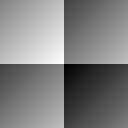``````laplacianOfGaussian stddev i j =
let r = fromIntegral (i*i + j*j)
x = (r / 2.0) / stddev
in (((-pi) / stddev) / stddev) * (1 - x) * (exp (-x))

*Main Data.Complex> let d2g = makeFilter 128 128 (laplacianOfGaussian 8) :: ComplexImage
*Main Data.Complex> d2g
< Image 128x128 >
*Main Data.Complex> display d2g
````````````fft :: (Image img, Image img', ComplexPixel (Pixel img), Pixel img' ~ Complex (Value (Pixel img))) => img -> img'
``````

Given an `Image` whose `Pixel`s can be converted to a complex value, `fft` returns an `Image` with `ComplexPixel`s representing its 2D discrete Fourier transform (DFT). Because the DFT is computed using the Fast Fourier Transform (FFT) algorithm, the number of rows and columns of the `Image` must both be powers of two, i.e., 2K where K is an integer.

For example,

``````*Main> let logFrog = magnitude . imageMap log . fft \$ frogpart
*Main> logFrog
< Image 128x128 >
*Main> display logFrog
``````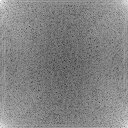``````*Main> fft d2g
< Image 128x128 >
*Main> display . fft \$ d2g
``````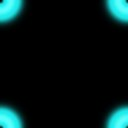``````gaussian variance i j =
let r = fromIntegral (i*i + j*j)
x = (r / (2*pi)) / variance
in exp (-x)

*Main> let g = makeFilter 128 128 (gaussian 8) :: GrayImage
*Main> display g
``````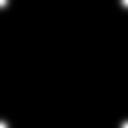``````*Main> fft g
< Image 128x128 >
*Main> display . fft \$ g
``````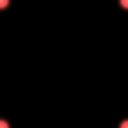``````ifft :: (Image img, Image img', ComplexPixel (Pixel img), Pixel img' ~ Complex (Value (Pixel img))) => img -> img'
``````

Given an `Image`, `ifft` returns a complex `Image` representing its 2D inverse discrete Fourier transform (DFT). Because the inverse DFT is computed using the Fast Fourier Transform (FFT) algorithm, the number of rows and columns of `Image` must both be powers of two, i.e., 2K where K is an integer.

For example,

``````*Main> ifft ((fft frogPart) * (fft d2g))

< Image 128x128 >
*Main> display \$ ifft ((fft frogPart) * (fft d2g))
``````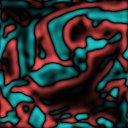``````*Main> ifft ((fft frogPart) * (fft g))
< Image 128x128 >
*Main> display \$ ifft ((fft frogPart) * (fft g))
``````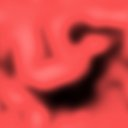``````realPart :: (Image img, Image img', ComplexPixel (Pixel img), Pixel img' ~ Value (Pixel img)) => img -> img'
``````

Given a complex `Image`, returns a real `Image` representing the real part of the `Image`.

For example,

``````*Main> let cosine = realPart signal :: GrayImage
*Main> cosine
< Image 128x128 >
*Main> display cosine
``````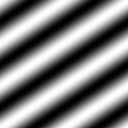``````*Main> display . realPart . ifft \$ (fft frogpart) * (fft d2g)
``````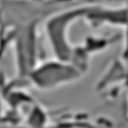``````*Main> display . realPart . ifft \$ (fft frogpart) * (fft g)
``````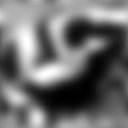``````imagPart :: (Image img, Image img', ComplexPixel (Pixel img), Pixel img' ~ Value (Pixel img)) => img -> img'
``````

Given a complex `Image`, `imagPart` returns a real `Image` representing the imaginary part of the `Image`.

For example,

``````*Main> let sine = imagPart signal :: GrayImage
*Main> sine
< Image 128x128 >
*Main> display sine
``````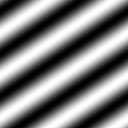``````complex :: (Image img, Image img', Pixel img' ~ C.Complex (Pixel img)) => img -> img -> img'
``````

Given an `Image` representing the real part of a complex `Image`, and an `Image` representing the imaginary part of a complex `Image`, `complex` returns a complex `Image`.

For example,

``````*Main> complex cosine sine :: ComplexImage
< Image 128x128 >
*Main> display (complex cosine sine :: ComplexImage)
````````````complexImageToRectangular :: (Image img, Image img', ComplexPixel (Pixel img), Pixel img' ~ Value (Pixel img)) => img -> (img', img')
``````

Given a complex `Image`, `complexImageToRectangular` returns a pair of real `Images` each representing a component of the complex `Image` (real, imaginary).

For example,

``````*Main> leftToRight' . complexImageToRectangular \$ signal
< Image 128x256 >
*Main> display . leftToRight' . complexImageToRectangular \$ signal
``````

![complesSignalToRectangular][]

``````magnitude :: (Image img, Image img', ComplexPixel (Pixel img), Pixel img' ~ Value (Pixel img)) => img -> img'
``````

Given a complex `Image`, returns a real `Image` representing the magnitude of the `Image`.

``````angle :: (Image img, ComplexPixel (Pixel img), Image img', Pixel img' ~ Value (Pixel img)) => img -> img'
``````

Given a complex `Image`, `angle` returns a real `Image` representing the angle of the `Image`.

For example,

``````*Main> angle signal
< Image 128x128 >
*Main> display (angle signal :: GrayImage)
``````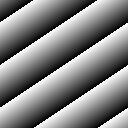``````complexImageToPolar :: (Image img, ComplexPixel (Pixel img), Image img', Pixel img' ~ Value (Pixel img)) => img -> (img', img')
``````

Given a complex `Image`, `complexImageToPolar` returns a pair of real `Image`s each representing the component (magnitude, phase) of the `Image`.

``````*Main> complexImageToPolar signal
(< Image 128x128 >,< Image 128x128 >)
*Main> display . leftToRight' . complexImageToPolar \$ signal
``````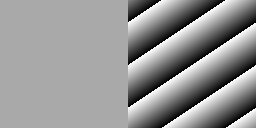``````(==) :: (==) :: Eq a => a -> a -> Bool
``````

`Image`s installed in the `Eq` type class (Boxed images) may be compared using the `(==)`. This returns `True` if and only if the `Image`s are of equal dimension and for each `Pixel` (i, j) in the two `Image`s are `(==)`.

``````(<) :: Ord a => a -> a -> Bool
``````

`Image`s installed in the `Ord` type class (Boxed images) may be compared using `(<)`. This returns `True` if and only if the `Image`s are of equal dimension and for each `Pixel` (i, j) in the two `Image`s are `(<)`.

``````(>) :: Ord a => a -> a -> Bool
``````

`Image`s installed in the `Ord` type class (Boxed images) may be compared using `(>)`. This returns `True` if and only if the `Image`s are of equal dimension and for each `Pixel` (i, j) in the two `Image`s are `(>)`.

``````(+) :: Num a => a -> a -> a
``````

Any two `Image`s installed in the `Num` type class (any two Boxed images) may be added if their dimensions match. For each (i, j) the resulting `Pixel` will be the sum of the `Pixel`s from the given `Image`s.

For example,

``````*Main> callisto <- readImage "images/callisto.pgm"
*Main> display callisto
``````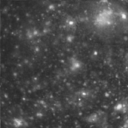``````*Main> ganymede <- readImage "images/ganymede.pgm"
*Main> display ganymede
``````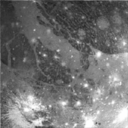``````*Main> callisto + ganymede
< Image 128x128 >
*Main> display \$ callisto + ganymede
``````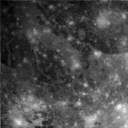``````(+.) :: (Num (Pixel i), Image i) => Pixel i -> i -> i
``````

Given a `Pixel` value and an `Image`, `(+.)` performs scalar addition to each `Pixel` in an `Image` and returns the result. Note: there is a flipped version of this function `(.+)`.

``````(-) :: Num a => a -> a -> a
``````

Any two `Image`s installed in the `Num` type class (any two Boxed images) may be subtracted if their dimensions match. For each (i, j) the resulting `Pixel` will be the difference of the two `Pixel`s from the given `Image`.

For example,

``````*Main> display \$ callisto - ganymede
``````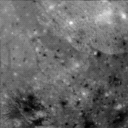``````(-.) :: (Num (Pixel i), Image i) => Pixel i -> i -> i
``````

Given a `Pixel` value and an `Image`, `(-.)` performs scalar subtraction to each `Pixel` in an `Image` and returns the result. Note: there is a flipped version of this function `(.-)`.

``````(*) :: Num a => a -> a -> a
``````

Any two `Image`s installed in the `Num` type class (any two Boxed images) may be multiplied if their dimensions match. For each (i, j) the resulting `Pixel` will be the product of the two `Pixel`s from the given `Image`s. For example,

``````*Main> display (callisto * ganymede)
``````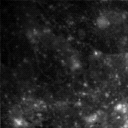``````(*.) :: (Num (Pixel i), Image i) => Pixel i -> i -> i
``````

Given a `Pixel` value and an `Image`, `(*.)` performs scalar multiplication to each `Pixel` in an `Image` and returns the result. Note: there is a flipped version of this function `(.*)`.

``````(/) :: Fractional a => a -> a -> a
``````

Any two `Image`s installed in the `Num` type class (any two Boxed images) may be divided if their dimensions match. For each (i, j) the resulting `Pixel` will be the quotient of the two `Pixel`s from the given `Image`s.

For example,

``````*Main> display (callisto / ganymede)
``````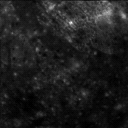``````(/.) :: (Fractional (Pixel i), Image i) => Pixel i -> i -> i
``````

Given a `Pixel` value and an `Image`, `(/.)` performs scalar division to each pixel in an `Image` and returns the result. Note: there is a flipped version of this function `(./)`.

``````arrayToImage :: Image img => Array (Int, Int) (Pixel img) -> img
``````

Given a two dimensional array of `Pixel` values indexed by pairs of `Int`s where the `fst` is the row and `snd` is the column, `arrayToImage` returns an `Image`.

For example,

``````*Main> let array = listArray ((0,0),(127,127)) [0..] :: Array (Int,Int) Double
*Main> arrayToImage array :: GrayImage
< Image 128x128 >
*Main> display (arrayToImage array :: GrayImage)
``````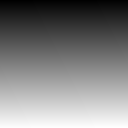``````imageToArray :: Image img => img -> Array (Int, Int) (Pixel img)
``````

Given img, `imageToArray` returns an two dimensional array of `Pixel` values indexed by pairs of `Int`s where the `fst` is the row and `snd` is the column.

``````*Main> let arr = listArray ((0,0),(2,2)) [0..] :: Array (Int, Int) Double
*Main> imageToArray (arrayToImage arr :: GrayImage)
array ((0,0),(2,2)) [((0,0),0.0),((0,1),1.0),((0,2),2.0),((1,0),3.0),((1,1),4.0),((1,2),5.0),((2,0),6.0),((2,1),7.0),((2,2),8.0)]
``````
``````(>.) :: (Ord (Pixel img), Image img, BinaryPixel (Pixel img)) => Pixel img -> img -> img
``````

Given a `Pixel` p and an `Image` img, return a `BinaryPixel` `Image` where the `Pixel` at (i, j) is on if p is greater than the corresponding `Pixel` in img at (i,j) and off otherwise. Note: there is a variation of `(>.)` named `(.>)` where the arguments are flipped.

``````*Main> stop <- readColorImage "images/stop.ppm"
*Main> display stop
````````````*Main> let (r,g,b) = colorImageToRGB stop
*Main> let binaryStop = (r + g + b) .> 400
*Main> display binaryStop
``````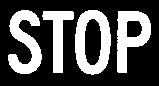``````(<.) :: (Ord (Pixel img), Image img, BinaryPixel (Pixel img)) => Pixel img -> img -> img
``````

Given a `Pixel` p and an `Image` img, return a `BinaryPixel` `Image` where the `Pixel` at (i, j) is on if p is less than the corresponding `Pixel` in img at (i,j) and off otherwise. Note: there is a variation of `(<.)` named `(.<)` where the arguments are flipped.

``````*Main> let (r,g,b) = colorImageToRGB stop
*Main> let invertBinaryStop = (r + g + b) .< 400
*Main> display invertBinaryStop
``````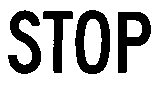``````(<~) :: (Ord (Pixel img), Image img) => Pixel img -> img -> Bool
``````

Given a `Pixel` value p and an `Image` img, return `True` if and only if all values in img are greater than p. Note: there is a variation of `(<~)` named`(~<)`where the arguments are flipped.

``````(.==.) :: (Eq (Pixel img), Image img, BinaryPixel (Pixel img)) => img -> img -> img
``````

Note to the reader, the documentation for the `(.==.)` is not correct. It will be updated shortly.

Given an `Image` with `Pixel`s, p, and a `Pixel`, c, returns an `Image` where each `Pixel` has the value 1 if and only if p = c and 0 otherwise. Note: there is a variation of `(==.)` named `(.==)` in which each `Pixel` in the `Image` is compared to a single specified `Pixel`.

``````shiftRight :: Image img => Int -> img -> img
shiftRight s img = makeImage (rows img) (cols img) shift where
shift r c = ref img r c' where
c' = let sum = c + s
in if sum < (cols img) then sum else sum - (cols img)
*Main> let binaryStop = (r + g + b) .> 400
*Main> display \$ (shiftRight 100 binaryStop)
````````````*Main> display \$ (shiftRight 100 binaryStop) .==. binaryStop
``````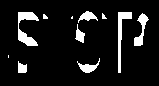``````normalize :: (Fractional (Pixel img), MaxMin (Pixel img), Image img) => img -> img
``````

Given img, `normalize` returns an image with the same dimensions where the values have been normalized to lie in the interval [0, 1].

``````shrink :: (Num a, Image img, ComplexPixel (Pixel img), Image img', Pixel img' ~ C.Complex (Value (Pixel img))) => a -> img -> img'
``````

Given a complex `Image` and a real positive number x, `shrink` returns a complex `Image` with the same dimensions. Let z be the value of the `Image` at location (i, j). The value of the complex result `Image` at location (i, j) is zero if |z| < x, otherwise the result has the same phase as z but the amplitude is decreased by x.

``````medianFilter :: (Ord (Pixel img), Image img) => Int -> Int -> img -> img
``````

Given two positive integers, m and n and a an `Image`, `medianFilter` returns an `Image` with the same dimensions where each `Pixel` (i, j) in `Image` is replaced by the `Pixel` with median value in the neighborhood of size m times n centered on (i, j).

``````*Main> let medianFilteredFrog = medianFilter 5 5 frog
*Main> display medianFilteredFrog
``````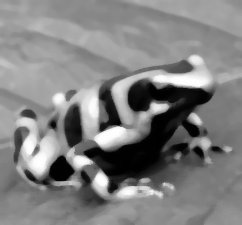``````imageFold :: Image img => (Pixel img -> b -> b) -> b -> img -> b
``````

Given a function of a `Pixel` to a value of type `b` which returns a value of type `b`, `imageFold` returns the value of type `b` which results from repeatedly applying the function to:

1. The result accumulated to this point. (initially the value of the first `Pixel`);
2. The value of the next `Pixel`.
``````matrixProduct :: (Num (Pixel img), Image img) => img -> img -> img
``````

Given an `Image` X1 and an `Image` X2, where the number of columns of X1 equals the number of rows of X2, `matrixProduct` returns an `Image` representing the matrix product of X1 and X2.

``````*Main> display (matrixProduct frogPart frogPart)
``````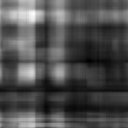``````imageMap :: (Image a, Image b) => (Pixel a -> Pixel b) -> a -> b
``````

Given a function of a `Pixel` value of type `a` to a `Pixel` value of type `b`, and an `Image` containing `Pixel` values of type `a`, `imageMap` returns an `Image` of type `b` where each `Pixel` in the result `Image` is the result of appyling the function to each `Pixel` in the given `Image`. Note: Boxed images are in typeclass `Functor` and `Applicative` it is recommended you use `fmap` instead of `imageMap` for Boxed images.

``````*Main> let img = imageMap ((-1) *) frog :: GrayImage
*Main> display img
``````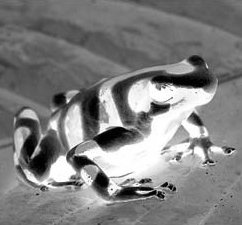``````readColorImage :: FilePath -> IO ColorImage
``````

Given the file path to a file containing an image stored in ASCII .ppm format, `readColorImage` reads the file and returns the `ColorImage`.

For example,

``````*Main> cacti <- readColorImage "images/cacti.ppm"
*Main> display cacti
````````````colorImageRed :: ColorImage -> GrayImage
``````

Given a `ColorImage`, `colorImageRed` returns a `GrayImage` representing the red color component.

For example,

``````*Main> let red = colorImageRed cacti
*Main> display red
``````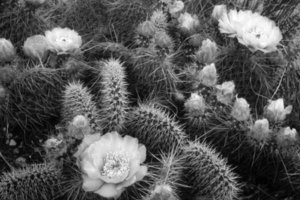``````colorImageGreen :: ColorImage -> GrayImage
``````

Given a `ColorImage`, `colorImageGreen` returns a `GrayImage` representing the green color component

For example,

``````*Main> let green = colorImageGreen cacti
*Main> display green
``````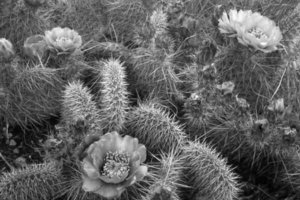``````colorImageBlue :: ColorImage -> GrayImage
``````

Given a `ColorImage`, `colorImageBlue` returns a `GrayImage` representing the blue color component

For example,

``````*Main> let blue = colorImageBlue cacti
*Main> display blue
``````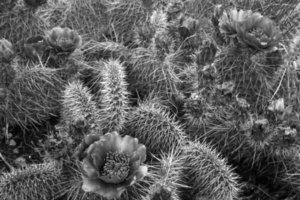``````rgbToColorImage :: (GrayImage, GrayImage, GrayImage) -> ColorImage
``````

Given a triple containing three `GrayImage`s each containing one of the color components (red, green, blue), `rgbToColorImage` returns a `ColorImage`.

``````*Main> display . rgbToColorImage \$ (red,green,blue)
````````````colorImageToRGB :: ColorImage -> (GrayImage, GrayImage, GrayImage)
``````

Given a `ColorImage`, `colorImageToRGB` returns a triple containing three `GrayImage`s each containing one of the color components (red, green, blue)

For example,

``````*Main> display . leftToRight' \$ colorImageToRGB cacti
``````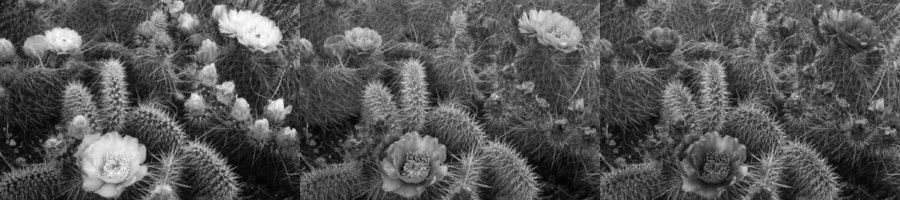``````colorImageToHSI :: ColorImage -> (GrayImage, GrayImage, GrayImage)
``````

Given a `ColorImage`, `colorImageToHSI` returns a triple containing three `GrayImage`s each containing one of the components (hue, saturation, intensity).

For example,

``````*Main> let (h,s,i) = colorImageToHSI cacti
*Main> display h
``````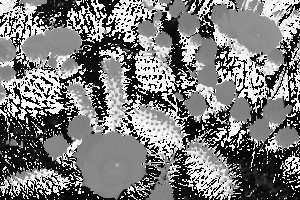``````*Main> display s
``````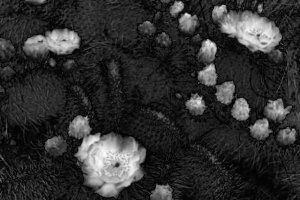``````*Main> display i
``````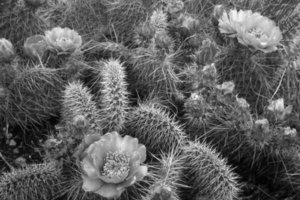``````hsiToColorImage :: (GrayImage, GrayImage, GrayImage) -> ColorImage
``````

Given a triple containing three `GrayImage`s each containing one of the color components (hue, saturation, intensity), `hsiToColorImage` returns a `ColorImage`.

For example,

``````*Main> display . hsiToColorImage \$ (h, s, i)
````````````makeHotImage :: GrayImage -> ColorImage
``````

Given a `GrayImage`, `makeHotImage` returns a `ColorImage` with the same dimensions. The R, G, B values of the result `ColorImage` at (i, j) are determined by using the value of the `GrayImage` at (i, j) to index three lookup tables. These lookup tables implement a false coloring scheme which maps small values to black, large values to white, and intermediate values to shades of red, orange, and yellow (in that order).

``````*Main> display . makeHotImage \$ frog
``````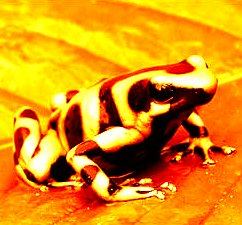``````dilate :: (Eq (Pixel img), Num (Pixel img), Image img, BinaryPixel (Pixel img)) => \[\[Pixel img\]\] -> img -> img
``````

Given a 2D list consisting solely of `Pixel`s representing a structuring element, and a `BinaryPixel` `Image`, `dilate` returns the morphological dilation of the `Image` with the structuring element. Note: There is a `dilate'` function that uses a default structuring element of [[1,1], [1,1]]. For example,

``````structure = [[0, 0, 1, 0, 0],
[0, 1, 1, 1, 0],
[1, 1, 1, 1, 1],
[0, 1, 1, 1, 0],
[0, 0, 1, 0, 0]]

*Main> display . dilate structure \$ binaryStop
````````````erode :: (Eq (Pixel img), Num (Pixel img), Image img, BinaryPixel (Pixel img)) => \[\[Pixel img\]\] -> img -> img
``````

Given a 2D list consisting solely of `Pixel`s representing a structuring element, and a `BinaryPixel` `Image`, `erode` returns the morphological erosion of the `Image` with the structuring element. Note: There is a `erode'` function that uses a default structuring element of [[1,1], [1,1]].

For example,

``````*Main> display . erode structure \$ binaryStop
````````````outline :: (Image img, BinaryPixel (Pixel img), Eq (Pixel img)) => img -> img
``````

Given an `Image`, `outline` returns an `Image` where edge `Pixel`s are set to the value on and non-edge `Pixel`s are set to the value off `Pixel` (i, j) is an edge `Pixel` if and only if its value is different than the value of either `Pixel` (i, j+1) or `Pixel` (i+1, j). Note: There is an `outline'` that allows the for the non-edge and edge `Pixel` values to be specified.

``````*Main> display . outline \$ binaryStop
``````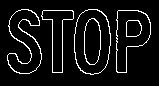``````label :: (Image img, BinaryPixel (Pixel img)) => img -> GrayImage
``````

Given a `BinaryPixel` `Image`, `label` returns an `Image` where `Pixel`s in distinct connected components (based on 4-neighbor connectivity) have distinct integer values. These values range from 1 to n where n is the number of connected components in `Image`.

``````*Main> display . makeHotImage . label \$ binaryStop
````````````distanceTransform :: (Image img, BinaryPixel (Pixel img)) => img -> GrayImage
``````

Given a `BinaryPixel` `Image`, `distanceTransform` returns an `Image` representing the 2D distance transform of the `Image`. The distance transform is accurate to within a 2% error for euclidean distance.

``````*Main> display . distanceTransform . dilate \$ binaryStop
``````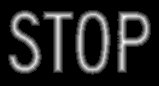``````open :: (Eq (Pixel img), Num (Pixel img), Image img, BinaryPixel (Pixel img)) => \[\[Pixel img\]\] -> img -> img
``````

Given a 2D list consisting solely of `Pixel`s representing a structuring element, and a `BinaryPixel` `Image`, `open` returns the morphological opening of the `Image` with the structuring element. Note: There is a version `open'` that uses the default structuring element [[1,1],[1,1]].

``````Main*> noise <- readColorImage "images/noise.ppm"
``````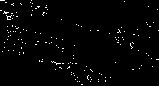``````Main*> let noisyStop = binaryStop ./=. noise
``````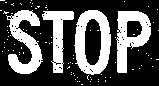``````Main*> display . open \$ noisyStop
``````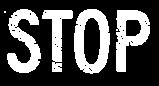``````close :: (Eq (Pixel img), Num (Pixel img), Image img, BinaryPixel (Pixel img)) => \[\[Pixel img\]\] -> img -> img
``````

Given a 2D list consisting solely of `Pixel`s representing a structuring element, and a `BinaryPixel` `Image`, `close` returns the morphological closing of the `Image` with the structuring element. Note: There is a version `close'` that uses the default structuring element [[1,1],[1,1]].

``````Main*>close [[1,1],[1,1]] noisyStop
``````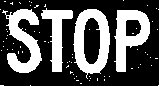``````areas :: (Image img, MaxMin (Pixel img), RealFrac (Pixel img)) => img -> V.Vector Double
``````

Given an `Image`, `areas` returns a `Vector` where the n-th component equals the number of `Pixel`s with value n. If the `Image` is the result of applying label to a `BinaryPixel` `Image`, then the `Vector` represents the areas of the connected-components of the `BinaryPixel` `Image`. If not, areas returns the histogram of the `Image`.

For example,

``````*Main> areas . label \$ binaryStop
fromList [9240.0,1154.0,1326.0,809.0,1145.0]
``````
``````perimeters :: (Image img, MaxMin (Pixel img), Pixel img ~ Double) => img -> V.Vector Double
``````

Given an `Image`, `perimeters` returns a `Vector` where the n-th component equals the number of `Pixel`s with value n which are adjacent to `Pixel`s of value 0 and the 0-th component equals the sum of the other components. If the `Image` is the result of applying label to a `BinaryPixel` `Image`, then the `Vector` represents the perimeters of the connected-components of the `BinaryPixel` `Image`.

For example,

``````*Main> perimeters . label \$ binaryStop
fromList [1082.0,312.0,326.0,184.0,260.0]
``````
``````centersOfMass :: (Image img, MaxMin (Pixel img), Pixel img ~ Double) => img -> [(Double, Double)]
``````

Given an `Image`, the result of applying label to a `BinaryPixel` `Image`, `centersOfMass` returns a `Vector` where the n-th component is a tuple representing the average row and column indices of `Pixel`s of the n-th connected-component of the `Image`.

For example,

``````*Main> centersOfMass . label \$ binaryStop
[(42.2686308492201,24.657712305025996),(41.74660633484163,92.20889894419307),(35.31025957972806,57.595797280593324),(35.583406113537116,129.9170305676856)]
``````
``````boundingBoxes :: (Image img, MaxMin (Pixel img), Pixel img ~ Double) => img -> [(Int, Int, Int, Int)]
``````

Given an `Image`, the result of applying label to a `BinaryPixel` `Image`, `boundingBoxes` returns a `Vector` where the n-th component is a four element tuple representing the minimum and maximum row and column indices of `Pixel`s of the n-th connected-component of the `Image`.

For example,

``````*Main> boundingBoxes . label \$ binaryStop
[(10,8,73,41),(10,75,74,110),(11,42,72,73),(11,117,72,150)]
``````